# 14.7: Aryl Halides

$$\newcommand{\vecs}{\overset { \rightharpoonup} {\mathbf{#1}} }$$ $$\newcommand{\vecd}{\overset{-\!-\!\rightharpoonup}{\vphantom{a}\smash {#1}}}$$$$\newcommand{\id}{\mathrm{id}}$$ $$\newcommand{\Span}{\mathrm{span}}$$ $$\newcommand{\kernel}{\mathrm{null}\,}$$ $$\newcommand{\range}{\mathrm{range}\,}$$ $$\newcommand{\RealPart}{\mathrm{Re}}$$ $$\newcommand{\ImaginaryPart}{\mathrm{Im}}$$ $$\newcommand{\Argument}{\mathrm{Arg}}$$ $$\newcommand{\norm}{\| #1 \|}$$ $$\newcommand{\inner}{\langle #1, #2 \rangle}$$ $$\newcommand{\Span}{\mathrm{span}}$$ $$\newcommand{\id}{\mathrm{id}}$$ $$\newcommand{\Span}{\mathrm{span}}$$ $$\newcommand{\kernel}{\mathrm{null}\,}$$ $$\newcommand{\range}{\mathrm{range}\,}$$ $$\newcommand{\RealPart}{\mathrm{Re}}$$ $$\newcommand{\ImaginaryPart}{\mathrm{Im}}$$ $$\newcommand{\Argument}{\mathrm{Arg}}$$ $$\newcommand{\norm}{\| #1 \|}$$ $$\newcommand{\inner}{\langle #1, #2 \rangle}$$ $$\newcommand{\Span}{\mathrm{span}}$$

Aryl halides have a halogen directly bonded to a carbon of an aromatic ring. Examples are bromobenzene, fluorobenzene, and 2,4-dichloromethylbenzene:Some of the methods by which alkyl halides are prepared do not work for aryl halides because it is difficult to form $$\ce{C}$$-halogen bonds at aromatic ring carbons by nucleophilic displacement reactions. The most common ways of forming $$\ce{C}_\text{aryl}$$-halogen bonds are by substitution of $$\ce{C}_\text{aryl} \ce{-H}$$ by electrophilic halogenating agents (e.g., $$\ce{Br_2}$$ or $$\ce{Cl_2}$$),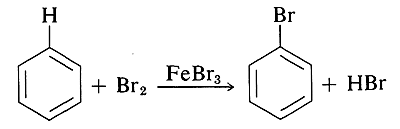and by replacement of $$\ce{C-NH_2}$$ by $$\ce{C}-$$halogen. These reactions are listed in Table 14-5 and will be discussed in more detail in Chapters 22 and 23.

## Nucleophilic Aromatic Displacement Reactions

The carbon-halogen bonds of aryl halides are like those of alkenyl halides in being much stronger than those of alkyl halides (see Table 4-6). The simple aryl halides generally are resistant to attack by nucleophiles in either $$S_\text{N}1$$ or $$S_\text{N}2$$ reactions (Table 14-6). However, this low reactivity can be changed dramatically by changes in the reaction conditions and the structure of the aryl halide. In fact, nucleophilic displacement becomes quite rapid (a) when the aryl halide is activated by substitution with strongly electron-attracting groups such as $$\ce{NO_2}$$, and (b) when very strongly basic nucleophilic reagents are used.

## Addition-Elimination Mechanism of Nucleophilic Substitution

Although the simple aryl halides are inert to the usual nucleophilic reagents, considerable activation is produced by strongly electron-attracting substituents provided these are located in either the ortho or para positions, or both. For example, the displacement of chloride ion from 1-chloro-2,4-dinitrobenzene by dimethylamine occurs readily in ethanol solution at room temperature. Under the same conditions chlorobenzene completely fails to react; thus the activating influence of the two nitro groups amounts to a factor of at least $$10^8$$:A related reaction is that of 2,4-dinitrofluorobenzene with the amino groups of peptides and proteins, and this reaction provides a means for analysis of the N-terminal amino acids in polypeptide chains. (See Section 25-7B.)

In general, the reactions of activated aryl halides closely resemble the $$S_\text{N}2$$-displacement reactions of aliphatic halides. The same nucleophilic reagents are effective (e.g., $$\ce{CH_3O}^\ominus$$, $$\ce{HO}^\ominus$$, and $$\ce{RNH_2}$$); the reactions are second order overall (first order in halide and first order in nucleophile); and for a given halide the more nucleophilic the attacking reagent, the faster the reaction. However, there must be more than a subtle difference in mechanism because an aryl halide is unable to pass through the same type of transition state as an alkyl halide in $$S_\text{N}2$$ displacements.

The generally accepted mechanism of nucleophilic aromatic substitution of aryl halides carrying activating groups involves two steps that are closely analogous to those briefly described in Section 14-4 for alkenyl and alkynyl halides. The first step involves attack of the nucleophile $$\ce{Y}^\ominus$$ at the carbon bearing the halogen substituent to form an intermediate carbanion $$4$$ (Equation 14-3). The aromatic system is destroyed on forming the anion, and the carbon at the reaction site changes from planar ($$sp^2$$ bonds) to tetrahedral ($$sp^3$$ bonds).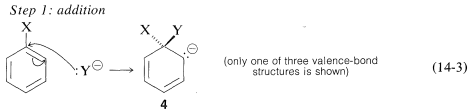In the second step, loss of an anion, $$\ce{X}^ominus$$ or $$\ce{Y}^\ominus$$, regenerates an aromatic system, and, if $$\ce{X}^\ominus$$ is lost, the overall reaction is nucleophilic displacement of $$\ce{X}$$ by $$\ce{Y}$$ (Equation 14-4).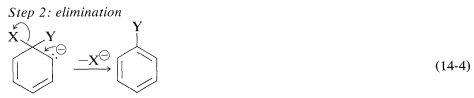In the case of a neutral nucleophilic reagent, $$\ce{Y}$$ or $$\ce{HY}$$, the reaction sequence would be the same except for the necessary adjustments in the charge of the intermediate:Why is this reaction pathway generally unfavorable for the simple aryl halides? The answer is that the intermediate $$4$$, which we can express as a hybrid of the valence-bond structures $$4a$$-$$4c$$, is too high in energy to be formed at any practical rate. Not only has $$4$$ lost the aromatic stabilization of the benzene ring, but its formation results in transfer of negative charge to the ring carbons, which themselves are not very electronegative:However, when strongly electron-attracting groups are located on the ring at the ortho-para positions, the intermediate anion is stabilized by delocalization of electrons from the ring carbons to more favorable locations on the substituent groups. As an example, consider the displacement of bromine by $$\ce{OCH_3}$$ in the reaction of 4-bromonitrobenzene and methoxide ion:The anionic intermediate formed by addition of methoxide ion to the aryl halide can be described by the valence-bond structures $$5a$$-$$5d$$. Of these structures $$5d$$ is especially important because in it the charge is transferred from the ring carbons to the oxygen of the nitro substituent:Substituents in the meta positions have much less effect on the reactivity of an aryl halide because delocalization of electrons to the substituent is not possible. No formulas can be written analogous to $$5c$$ and $$5d$$ in which the negative charges are both on atoms next to positive nitrogen, $$\overset{\ominus}{\ce{C}} \overset{\oplus}{\ce{-N}-} \overset{\ominus}{\ce{O}}$$ and $$\overset{\ominus}{\ce{O}} \overset{\oplus}{\ce{-N}-} \overset{\ominus}{\ce{O}}$$,In a few instances, stable compounds resembling the postulated reaction intermediate have been isolated. One classic example is the complex $$7$$ (isolated by J. Meisenheimer), which is the product of the reaction of either the methyl aryl ether 6$$6$$ with potassium ethoxide, or the ethyl aryl ether $$8$$ and potassium methoxide: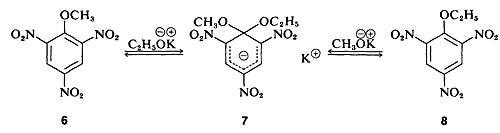## Elimination-Addition Mechanism of Nucleophilic Aromatic Substitution. Arynes

The reactivities of aryl halides, such as the halobenzenes, are exceedingly low toward nucleophilic reagents that normally effect displacements with alkyl halides and activated aryl halides. Substitutions do occur under forcing conditions of either high temperatures or very strong bases. For example, chlorobenzene reacts with sodium hydroxide solution at temperatures around $$340^\text{o}$$ and this reaction was once an important commercial process for the production of benzenol (phenol):In addition, aryl chlorides, bromides, and iodides can be converted to areneamines $$\ce{ArNH_2}$$ by the conjugate bases of amines. In fact, the reaction of potassium amide with bromobenzene is extremely rapid, even at temperatures as low as $$-33^\text{o}$$ with liquid ammonia as solvent:However, displacement reactions of this type differ from the previously discussed displacements of activated aryl halides in that rearrangement often occurs. That is, the entering group does not always occupy the same position on the ring as that vacated by the halogen substituent. For example, the hydrolysis of 4-chloromethylbenzene at $$340^\text{o}$$ gives an equimolar mixture of 3- and 4-methylbenzenols: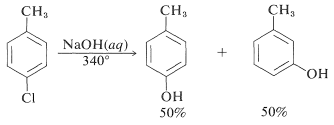Even more striking is the exclusive formation of 3-methoxybenzenamine in the amination of 2-chloromethoxybenzene. Notice that this result is a violation of the principle of least structural change (Section 1-1H):The mechanism of this type of reaction has been studied extensively, and much evidence has accumulated in support of a stepwise process, which proceeds first by base-catalyzed elimination of hydrogen halide $$\left( \ce{HX} \right)$$ from the aryl halide - as illustrated below for the amination of bromobenzene:

EliminationThe product of the elimination reaction is a highly reactive intermediate $$9$$ called benzyne, or dehydrobenzene, which differs from benzene in having two less hydrogen and an extra bond between two ortho carbons. Benzyne reacts rapidly with any available nucleophile, in this case the solvent, ammonia, to give an addition product: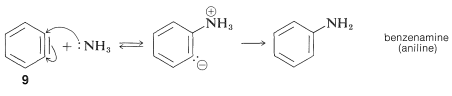The rearrangements in these reactions result from the attack of the nucleophile at one or the other of the carbons of the extra bond in the intermediate. With benzyne the symmetry is such that no rearrangement would be detected. With substituted benzynes isomeric products may result. Thus 4-methylbenzyne, $$10$$, from the reaction of hydroxide ion with 4-chloro-1-methylbenzene gives both 3- and 4-methylbenzenols:In the foregoing benzyne reactions the base that produces the benzyne in the elimination step is derived from the nucleophile that adds in the addition step. This need not always be so, depending on the reaction conditions. In fact, the synthetic utility of aryne reactions depends in large part of the success with which the aryne can be generated by one reagent but captured by another. One such method will be discussed in Section 14-10C and involves organometallic compounds derived from aryl halides. Another method is to generate the aryne by thermal decomposition of a 1,2-disubstituted arene compound such as $$11$$, in which both substituents are leaving groups - one leaving with an electron pair, the other leaving without:When $$11$$ decomposes in the presence of an added nucleophile, the benzyne intermediate is trapped by the nucleophile as it is formed. Or, if a conjugated diene is present, benzyne will react with it by a [4 + 2] cycloaddition. In the absence of other compounds with which it can react, benzyne will undergo [2 + 2] cycloaddition to itself:## Uses for Aryl Halogen Compounds

As with most organic halides, aryl halides most often are synthetic intermediates for the production of other useful substances. For example, chlorobenzene is the starting aryl halide for the synthesis of DDT; it also is a source of benzenol (phenol, Section 14-6C) which, in turn, has many uses (Section 26-1).

Several aromatic chloro compounds are used extensively as insecticides, herbicides, fungicides, and bactericides. They also have acquired much notoriety because in some instances their indiscriminate usage has led to serious problems. For example, hexachlorophene is an external bactericide that until recently was used in cosmetic preparations such as soaps, deodorants, and so on. Its use has been discontinued because of compelling evidence that it can be absorbed through the skin in amounts that are dangerous, if not lethal, for infants and small children.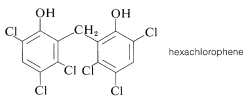Other pesticides, notably DDT and the herbicides 2,4-D and 2,4,5-T have been partially banned for different reasons.